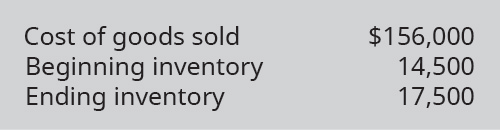# Compute Westtown Company’s (A) inventory turnover ratio and (B) number of days’ sales in inventory ratio, using the following information.FindFindarrow_forward

### Principles of Accounting Volume 1

19th Edition
OpenStax
Publisher: OpenStax College
ISBN: 9781947172685

#### Solutions

Chapter
SectionFindFindarrow_forward

### Principles of Accounting Volume 1

19th Edition
OpenStax
Publisher: OpenStax College
ISBN: 9781947172685
Chapter 10, Problem 16EB
Textbook Problem
1 views

## Compute Westtown Company’s (A) inventory turnover ratio and (B) number of days’ sales in inventory ratio, using the following information.To determine

(a)

Concept introduction:

Inventory Turnover Ratio:

Inventory Turnover Ratio measures the efficiency of the company in converting its inventory into sales. It is calculated by dividing the Cost of goods sold by Average inventory. The formula of the Inventory Turnover Ratio is as follows:

Inventory Turnover Ratio=Cost of goods soldAverage inventory

Note: Average inventory is calculated with the help of following formula:

Average inventory=(Beginning inventory + Ending inventory)2

Day’s sales in inventory:

Days sales in inventory represent the number of days the inventory waits for the sale. It is calculated using the following formula:

Day Sales in Inventory =  Inventory *365Cost of Goods Sold

To calculate:

The Inventory Turnover Ratio

### Explanation of Solution

The Inventory Turnover Ratio is calculated as follows:

...
 Cost of Goods Sold (A) $156,000 Beginning Inventory (B)$ 14,500
To determine

(b)

Concept introduction:

Inventory Turnover Ratio:

Inventory Turnover Ratio measures the efficiency of the company in converting its inventory into sales. It is calculated by dividing the Cost of goods sold by Average inventory. The formula of the Inventory Turnover Ratio is as follows:

Inventory Turnover Ratio=Cost of goods soldAverage inventory

Note: Average inventory is calculated with the help of following formula:

Average inventory=(Beginning inventory + Ending inventory)2

Day’s sales in inventory:

Days sales in inventory represent the number of days the inventory waits for the sale. It is calculated using the following formula:

Day Sales in Inventory =  Inventory *365Cost of Goods Sold

To calculate:

Number of Day’s sales in inventory ratio

### Still sussing out bartleby?

Check out a sample textbook solution.

See a sample solution

#### The Solution to Your Study Problems

Bartleby provides explanations to thousands of textbook problems written by our experts, many with advanced degrees!

Get Started

Find more solutions based on key concepts
How are inflation and unemployment related in the short run?

Essentials of Economics (MindTap Course List)

What are the steps in entering international markets?

Foundations of Business (MindTap Course List)

EXCHANGE GAINS AND LOSSES You are the vice president of International InfoXchange, headquartered in Chicago, Il...

Fundamentals of Financial Management, Concise Edition (with Thomson ONE - Business School Edition, 1 term (6 months) Printed Access Card) (MindTap Course List)

What is a database?

Accounting Information Systems

How do unions affect the natural rate of unemployment?

Principles of Macroeconomics (MindTap Course List)

Describe a firms macro environment.

Principles of Management